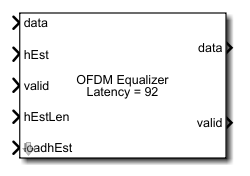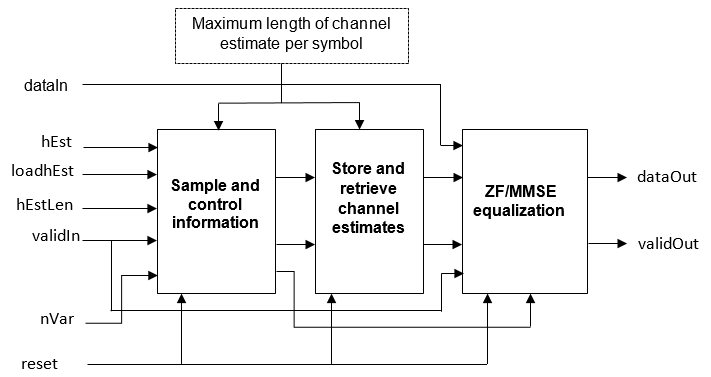# OFDM Equalizer

Equalize OFDM data using channel estimates

Since R2021a

•Libraries:
Wireless HDL Toolbox / Modulation

## Description

The OFDM Equalizer block equalizes the OFDM data using channel estimates. The block supports zero-forcing (ZF) and minimum mean square error (MMSE) algorithms for channel equalization in the frequency domain. The block accepts data symbols, estimated channel (hEst), and the estimated channel length per symbol (hEstLen) data ports and valid and loadhEst control ports. The block outputs an equalized data port and a valid control port.

You can use this block to equalize channel effects in different communications standards, such as long term evolution (LTE) , 5G new radio (NR) standard TS 38.212 , and wireless local area network (WLAN) .

The block provides an interface and architecture suitable for HDL code generation and hardware deployment.

## Ports

### Input

expand all

OFDM data, specified as a complex-valued scalar.

The software supports `double` and `single` data types for simulation, but not for HDL code generation.

Data Types: `single` | `double` | `int8` | `int16` | `int32` | `signed fixed point`

Channel estimated data, specified as a complex-valued scalar.

The input data type must be `fixdt(1,k,m)`, where k is less than or equal to 30, and m is less than k.

The software supports `double` and `single` data types for simulation, but not for HDL code generation.

Data Types: `single` | `double` | `int8` | `int16` | `fixed point`

Control to indicate valid input data, specified as a Boolean scalar.

This port is a control signal that indicates when the input data and hEst port values are valid. When this value is `1`, the block captures the values from the data and hEst input ports. When this value is `0`, the block ignores the values on the data and hEst input ports.

Data Types: `Boolean`

Length of the estimated channel per symbol, specified as a scalar in the range from 2 to 65,536.

To support the minimum number of subcarriers per symbol, this data type must be `fixdt(0,k,0)`, where k is greater than or equal to 2.

The software supports `double` and `single` data types for simulation, but not for HDL code generation.

Data Types: `single` | `double` | `int8` | `int16` | `int32` | `fixed point`

Channel estimates control, specified as a Boolean scalar.

When this value is `1`, the block loads the channel estimates until the length of the channel estimate specified by the hEstLen input port. hEstLen is sampled at loadhEst.

When this value is `0`, and the input is `1`, the block performs equalization with the previously sampled hEstLen input and the stored hEst input values. If the previously sampled hEstLen value is not available, the block performs equalization with the instantaneous inputs data, hEst, and nVar. For more information, see Algorithms.

Data Types: `Boolean`

Noise variance, specified as a scalar.

When the input valid is `1`, the block samples the nVar port. This value must be of data type `fixdt(0,k,m)`, where k is less than or equal to 16, and m is less than or equal to k.

The software supports `double` and `single` data types for simulation, but not for HDL code generation.

#### Dependencies

To enable this port, set the Equalization method parameter to `MMSE`.

Data Types: `single` | `double` | `uint8` | `uint16` | `fixed point`

Reset internal states of the block to start with new data, specified as a scalar.

#### Dependencies

To enable this port, select the Enable reset input port parameter.

Data Types: `Boolean`

### Output

expand all

Complex output data, returned as a scalar. The output data type is the same as the input data type.

Data Types: `single` | `double` | `int8` | `int16` | `int32` | `signed fixed point`

Indicates valid output data, returned as a Boolean scalar.

This port is a control signal that indicates when the data output port is valid. When the data samples are available on the data output port, the block sets this output valid value to `1`.

Data Types: `Boolean`

## Parameters

expand all

Specify the maximum length of the channel estimate per symbol.

To support the minimum number of subcarriers per symbol, which is 2, the data type of the hEstLen input must be `fixdt(0,k,0)`, where k is greater than or equal to 2.

Select this parameter to enable the reset input port.

## Algorithms

expand all

The OFDM Equalizer block supports ZF and MMSE algorithms for channel equalization in the frequency domain. The block stores the estimated channel information to equalize the OFDM symbols and generates the equalized output using these algorithms.

The OFDM Equalizer block operation sequence is implemented using these subsystem blocks: Sample and control information, Store and retrieve channel estimates, and ZF/MMSE equalization. This figure shows these blocks.The Sample and control information block samples and validates the hEstLen input based on the loadhEst input signal, validates the hEst and nVar inputs based on the validIn input signal, and outputs the sampled hEstOut output, nVarOut output, and the control information signals that are used in storing and retrieving channel information. The Store and retrieve channel estimates block stores and retrieves the channel using RAM and switches. The ZF/MMSE Equalization block performs ZF or MMSE equalization using these equations. The nVar input port is available when you set the Equalization method parameter to `MMSE`.

• ZF Algorithm:

`$dataOu{t}_{p}=\text{​}\text{\hspace{0.17em}}\left(hEs{t}_{p}{}^{*}\text{\hspace{0.17em}}×\text{\hspace{0.17em}}\text{\hspace{0.17em}}dataI{n}_{p}\right)\text{\hspace{0.17em}}/\text{\hspace{0.17em}}|hEs{t}_{p}{|}^{2\text{\hspace{0.17em}}}$`

• MMSE Algorithm:

`$dataOu{t}_{p}=\text{​}\text{\hspace{0.17em}}\left(hEs{t}_{p}{}^{*}\text{\hspace{0.17em}}\text{​}\text{​}×\text{\hspace{0.17em}}\text{\hspace{0.17em}}dataI{n}_{p}\right)/\text{\hspace{0.17em}}\left(|hEs{t}_{p}{|}^{2\text{\hspace{0.17em}}}\text{\hspace{0.17em}}+\text{\hspace{0.17em}}\text{\hspace{0.17em}}nVa{r}_{p}\right)$`

In these equations,

• dataIn is the demodulated output provided as an input to the block

• hEst is the estimated channel

• hEst* is the Hermitian of the estimated channel

• dataOut is the equalized output

• nVar is the noise variance

• p is equal to 0, 1, …. NSPS, where NSPS is the number of subcarriers per symbol.

This figure shows a sample block operation when you set the Equalization method parameter to `ZF`.In this figure, you can see three symbols (Symbol 1, Symbol 2, and Symbol 3) are input to the dataIn port. When the validIn input is `1` (high) and the loadHest input is `1` (high), the block samples the hEstlLen input value, which is `72` in this example. Based on the hEstlLen value, for Symbol 1, the block provides the equalized output for the instantaneous hEst input values. When the loadHest value changes to `0` (low), the block stores the hEst values and provides the equalized output for Symbol 2 based on the stored hEst values. The hEstLen value remains the same until the loadHest changes to `0` (low). Similarly, for Symbol 3, the block provides the equalized output for the instantaneous hEst values based on the hEstlLen value, which is `52` in this example.

 3GPP TS 36.211 version 14.2.0 Release 14. "Physical channels and modulation." LTE - Evolved Universal Terrestrial Radio Access (E-UTRA).

 3GPP TS 38.212. “NR; Multiplexing and Channel Coding.” 3rd Generation Partnership Project; Technical Specification Group Radio Access Network.

 "Wireless LAN Medium Access Control (MAC) and Physical layer (PHY) Specifications." IEEE Std 802.11 – 2012.